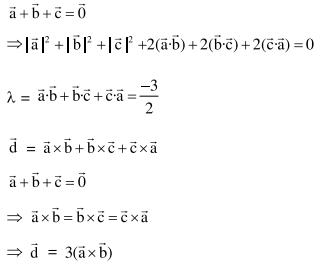Deepak Scored 45->99%ile with Bounce Back Crack Course. You can do it too!

# Prove the following

Question:

Let $\overrightarrow{\mathrm{a}}, \overrightarrow{\mathrm{b}}$ and $\overrightarrow{\mathrm{c}}$ be three units vectors such that $\overrightarrow{\mathrm{a}}+\overrightarrow{\mathrm{b}}+\overrightarrow{\mathrm{c}}=\overrightarrow{0}$. If $\lambda=\overrightarrow{\mathrm{a}} \cdot \overrightarrow{\mathrm{b}}+\overrightarrow{\mathrm{b}} \cdot \overrightarrow{\mathrm{c}}+\overrightarrow{\mathrm{c}} \cdot \overrightarrow{\mathrm{a}} \quad$ and $\overrightarrow{\mathrm{d}}=\overrightarrow{\mathrm{a}} \times \overrightarrow{\mathrm{b}}+\overrightarrow{\mathrm{b}} \times \overrightarrow{\mathrm{c}}+\overrightarrow{\mathrm{c}} \times \overrightarrow{\mathrm{a}}$, then the ordered pair, $(\lambda, \overrightarrow{\mathrm{d}})$ is equal to:

1. $\left(-\frac{3}{2}, 3 \vec{a} \times \vec{b}\right)$

2. $\left(-\frac{3}{2}, 3 \overrightarrow{\mathrm{c}} \times \overrightarrow{\mathrm{b}}\right)$

3. $\left(\frac{3}{2}, 3 \overrightarrow{\mathrm{b}} \times \overrightarrow{\mathrm{c}}\right)$

4. $\left(\frac{3}{2}, 3 \overrightarrow{\mathrm{a}} \times \overrightarrow{\mathrm{c}}\right)$

Correct Option: 1

Solution: